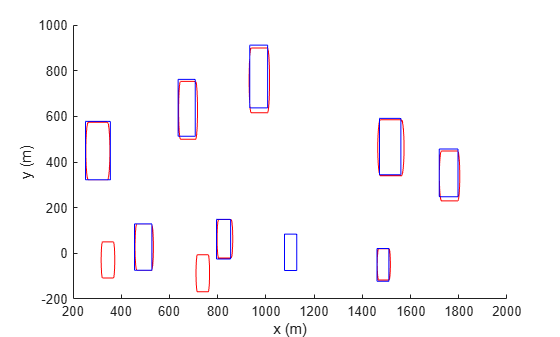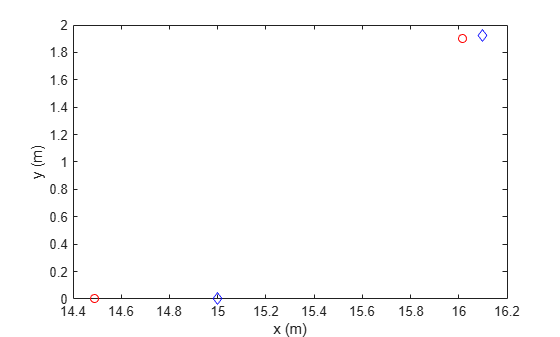# trackCLEARMetrics

CLEAR multi-object tracking metrics

Since R2023a

## Description

The `trackCLEARMetrics` object implements the Classification of Events, Activities, and Relationships (CLEAR) Multi-Object Tracking (MOT) metrics, which evaluate tracking performance by comparing tracks with ground truth. Other than CLEAR, the object also outputs Mostly-Tracked, Partially-Tracked, and Mostly-Lost MOT metrics. You can set the similarity method to intersection over union, Euclidean distance, and a custom method. The object uses the similarity method to compare tracks with truth.

## Creation

### Syntax

``metric = trackCLEARMetrics``
``metric = trackCLEARMetrics(Name=Value)``

### Description

````metric = trackCLEARMetrics` creates a default `trackCLEARMetrics` object. Use the `evaluate` object function to obtain various metrics.```

example

````metric = trackCLEARMetrics(Name=Value)` specifies properties using name-value arguments. Unspecified properties have defaults values.```

## Properties

expand all

Similarity threshold to assign tracks to detections, specified as a nonnegative scalar. The metric matches a track to a truth if their similarity is greater than or equal to the specified `SimilarityThrehold` property. The metric calculates the similarity between a track and a truth based on the similarity method specified in the `SimilarityMethod` property.

Method to calculate similarity between tracks and truths, specified as one of these values:

• `"IoU2d"` — The metric calculates the similarity based on the intersection over union for 2-D axis-aligned rectangles. When you specify this value, you must also specify the `BoundingBox` field for the `tracks` and `truths` inputs when evaluating the metric using the `evaluate` object function. See the input arguments of `evaluate` for more details.

• `"Euclidean"` — The metric calculates the similarity based on the Euclidean distances between tracks and truths as well as the scale defined in the `EuclideanScale` property. When you specify this value, you must also specify the `Position` field for the `tracks` and `truths` inputs when evaluating the metric using the `evaluate` object function. The position values can be 1-D, 2-D, or 3-D. See the input arguments of `evaluate` for more details.

• `"Custom"` — The metric calculates the similarity based on a custom similarity function defined in the `CustomSimilarityFcn` property.

Note

You can specify this property only when creating the object.

Data Types: `char` | `string`

Scale for Euclidean similarity, specified as a positive scalar. The metric calculates the similarity between a track and a truth as:

`$sim=\mathrm{max}\left(0,1-\frac{{d}_{E}}{{d}_{S}}\right)$`

where

• sim is the calculated similarity.

• dE is the Euclidean distance between a track and a truth.

• dS is the Euclidean scale.

#### Dependencies

To enable this property, set the `SimilarityMethod` property to `"Euclidean"`.

Custom similarity function to use to calculate the similarity value between tracks and truths returned from the `trackCLEARMetrics` object. The function must use this syntax:

`similarities = customFcn(tracks,truths)`
The `tracks` and `truths` are a track list and truth list of the same time stamp. Specifically,

• `tracks` — Track list, returned by the `trackCLEARMetrics` object as an N-element array of track structures. The track structures are in the same format as the `tracks` input of the `evaluate` object function except that these tracks are of the same time.

• `truths` — Truth list, returned by the `trackCLEARMetrics` object as an M-element array of truth structures. The track structures are in the same format as the `truths` input of the `evaluate` object function except that these truths are of the same time.

• `similarities` — Similarity values between tracks and truths, returned as an N-by-M matrix of scalars in range of [`0 1`]. The (i,j) element of the matrix represents the similarity between the i-th track in `tracks` and the j-th truth in `truths`.

Note

You can specify this property only when creating the object.

#### Dependencies

To enable this property, set the `SimilarityMethod` property to `"Custom"`.

## Object Functions

 `evaluate` Evaluate metrics on tracks and truths

## Examples

collapse all

Create a `trackCLEARMetrics` object and set the `SimilarityThreshold` property to `"0.8". `

`metric = trackCLEARMetrics(SimilarityThreshold=0.8);`

Load a tracking dataset consisting of truths and tracks.

`load("trackCLEARData.mat","tracks","truths");`

Visualize tracks in red and truths in blue.

```figure for t = 0:0.5:10 % Extract tracks at a certain time stamp. tracks_t = tracks([tracks.Time] == t); % Extract turths at a certain time stamp. truths_t = truths([truths.Time] == t); cla; % Clean plotting in axes if any. for j=1:numel(tracks_t) posTrack = tracks_t(j).BoundingBox; posTrack(2) = posTrack(2)-posTrack(4); rectangle(Position=posTrack,EdgeColor="r",Curvature=[0.2 1]); end for j=1:numel(truths_t) posTruth = truths_t(j).BoundingBox; posTruth(2) = posTruth(2)-posTruth(4); rectangle(Position=posTruth,EdgeColor="b"); end xlabel("x (m)") ylabel("y (m)") pause(0.2) % Pause the animation 0.2 seconds for each time stamp. end```Evaluate the CLEAR MOT metrics.

`metricTable = evaluate(metric,tracks,truths)`
```metricTable=1×12 table MOTA (%) MOTP (%) Mostly Tracked (%) Partially Tracked (%) Mostly Lost (%) False Positive False Negative Recall (%) Precision (%) False Track Rate ID Switches Fragmentations ________ ________ __________________ _____________________ _______________ ______________ ______________ __________ _____________ ________________ ___________ ______________ 27.737 85.492 50 40 10 56 43 68.613 62.667 3.7333 0 16 ```

Create a `trackCLEARMetrics` object. Set the `SimilarityMethod` property to `"Euclidean"` and set the `EuclideanScale` property to `2. `

`metric = trackCLEARMetrics(SimilarityMethod="Euclidean",EuclideanScale=2);`

Load a tracking dataset consisting of tracks and truths.

`load("trackCLEAREuclideanData.mat","tracks","truths");`

Visualize tracks in red circles and truths in blue diamonds.

```figure for t = 0:0.5:10 % Extract tracks at a certain time stamp. tracks_t = tracks([tracks.Time] == t); % Extract truths at a certain time stamp. truths_t = truths([truths.Time] == t); hold off; for j=1:numel(tracks_t) position = tracks_t(j).Position; plot(position(1),position(2),"ro") hold on; end for j=1:numel(truths_t) position = truths_t(j).Position; plot(position(1),position(2),"bd") end xlabel("x (m)") ylabel("y (m)") pause(0.2) % Pause the animation 0.2 seconds for each time stamp. end```Evaluate the CLEAR MOT metrics.

`metricTable = evaluate(metric,tracks,truths)`
```metricTable=1×12 table MOTA (%) MOTP (%) Mostly Tracked (%) Partially Tracked (%) Mostly Lost (%) False Positive False Negative Recall (%) Precision (%) False Track Rate ID Switches Fragmentations ________ ________ __________________ _____________________ _______________ ______________ ______________ __________ _____________ ________________ ___________ ______________ 57.5 80.934 50 50 0 3 14 65 89.655 0.14286 0 1 ```

Create a `trackCLEARMetrics` object. Set the `SimilarityMethod` property to `"Custom"` and set the `CustomSimilarityFcn` property to `@mySimilarityFcn. `

`metric = trackCLEARMetrics(SimilarityMethod="Custom",CustomSimilarityFcn=@mySimilarityFcn);`

Load a tracking dataset consisting of tracks and truths.

`load("trackCLEAREuclideanData.mat","tracks","truths");`

Visualize tracks in red circles and truths in blue diamonds.

```figure for t = 0:0.5:10 % Extract tracks at a certain time stamp. tracks_t = tracks([tracks.Time] == t); % Extract turths at a certain time stamp. truths_t = truths([truths.Time] == t); hold off; for j=1:numel(tracks_t) position = tracks_t(j).Position; plot(position(1),position(2),"ro") hold on; end for j=1:numel(truths_t) position = truths_t(j).Position; plot(position(1),position(2),"bd") end xlabel("x (m)") ylabel("y (m)") pause(0.2) % Pause the animation 0.2 seconds for each time stamp. end```Evaluate the CLEAR MOT metrics.

`metricTable = evaluate(metric,tracks,truths)`
```metricTable=1×12 table MOTA (%) MOTP (%) Mostly Tracked (%) Partially Tracked (%) Mostly Lost (%) False Positive False Negative Recall (%) Precision (%) False Track Rate ID Switches Fragmentations ________ ________ __________________ _____________________ _______________ ______________ ______________ __________ _____________ ________________ ___________ ______________ 72.5 95.397 50 50 0 0 11 72.5 100 0 0 1 ```

Custom Similarity Function

```function sims = mySimilarityFcn(tracks,truths) numTracks = numel(tracks); numTruths = numel(truths); sims = NaN(numTracks,numTruths); for i = 1:numTracks for j = 1:numTruths pTrack = tracks(i).Position; pTruth = truths(i).Position; sims(i,j) = max(0,1-norm(pTrack-pTruth)/norm(pTruth)); end end end```

## Algorithms

The `trackCLEARMetrics` object implements the CLEAR algorithms and takes these steps to calculate various metrics based on a list of tracks (T) and truths (R).

1. First, the object loops over each time step tk, k = 1, …, N, derived from the time in the list of truths.

1. The object extracts all the tracks and truths at time tk as Tk and Rk, respectively, from the track list (T) and truth list (R).

2. The object calculates the similarity scores between tracks in Tk and truths in Rk based on the specified similarity method.

3. Using the calculated similarities at tk and the matching history at tk-1, the object forms a cost matrix for matching between Tk and Rk. After that, the object matches between Tk and Rk based on the Hungarian assignment algorithm and the similarity threshold.

There are three types of results from the matching:

• True match — A match that associates a valid track in Tk to a valid truth in Rk.

• False positive — A match in which a track is not associated to any truths.

• False negative — A match in which a truth is not associated to any tracks.

4. The object increments the number of true matches, false positives, and false negatives, as well as the sum of all true matches similarity scores across timestamps.

5. Using the true matches of the previous timestamp, the object also increments the number of track switches and track fragmentations. A track switch occurs if a true object is matched in both the current and previous timestamps and the identity of the matched track changes across these two timestamps. A track fragmentation occurs if a true object has a true match in the current timestamp but does not have a true match in the previous timestamp.

2. Next, the object calculates metrics based on various values cumulated during the loop. In particular, it calculates the multiple object tracking accuracy (MOTA) , the multiple object tracking precision (MOTP), the Precision, and the Recall metrics as follows:

• MOTA metric:

`$\text{MOTA}=1-\frac{{n}_{fp}+{n}_{fn}+{n}_{s}}{{n}_{truths}},$`

where ntruths is the total number of truth occurrences across all time steps, nfp is the total number of false positives, nfn is the total number of false negatives, and ns is the total number of switches.

• MOTP:

`$\text{MOTP}=\frac{1}{{n}_{t}}\sum _{i=1}^{{n}_{t}}{s}_{i},$`

where nt is the total number of true matches across all time stamps and si is the similarity score of the i-th true match.

• Precision:

`$\text{Precision}=\frac{{n}_{t}}{{n}_{t}+{n}_{fp}},$`

where nt is the total number of true matches and nfp is the total number of false positives.

• Recall:

`$\text{Recall}=\frac{{n}_{t}}{{n}_{t}+{n}_{fn}},$`

where nt is the total number of true matches and nfn is the total number of false negatives.

The definitions for all the metrics are given in the `results` argument of the `evaluate` object function.

 Bernardin, Keni, and Rainer Stiefelhagen. “Evaluating Multiple Object Tracking Performance: The CLEAR MOT Metrics.” EURASIP Journal on Image and Video Processing, vol. 2008, 2008, pp. 1–10.

 Li, Yuan, et al. “Learning to Associate: HybridBoosted Multi-Target Tracker for Crowded Scene.” 2009 IEEE Conference on Computer Vision and Pattern Recognition, IEEE, 2009, pp. 2953–60.

 Luiten, Jonathon, et al. “HOTA: A Higher Order Metric for Evaluating Multi-Object Tracking.” International Journal of Computer Vision, vol. 129, no. 2, Feb. 2021, pp. 548–78.